# 5.3 Gradient Based Optimization Methods

Gradient based optimization strategies iteratively search a minimum of a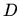dimensional target function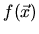. The target function is thereby approximated by a terminated Taylor series expansion around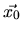: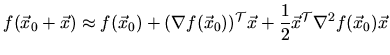The actual optimization is performed iteratively. After an initial value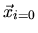was chosen and the target function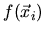was computed, the following loop tries to achieve a minimum:

1. Check the convergence criterion of; terminate with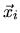as the solution if fulfilled.
2. Compute a direction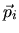and a step width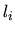3. Evaluate the new point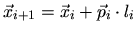; compute the target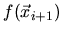and go to step 1.

To compute the step direction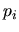a linear approximation (first order) of the target function can be used: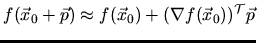which results in the step direction: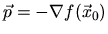This is called the steepest descent method. A second order approach uses a quadratic approximation: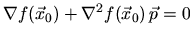This is referred to as Newton's direction method.

To compute the step width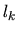the parameter vector of the next iteration is calculated by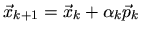The parameter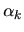denotes the step width and is chosen depending on the used optimization algorithm.

For an analytically given target function the first and second order derivatives can directly be transferred into a computer program, however if no explicit formula can be defined, the target is computed numerically by means of a simulation. In this case approximations for the derivatives are necessary. In the following the approximation of a univariate target function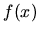is given. For multivariate target functions the finite difference approximation is applied for each dimension.

The performance of a gradient based method strongly depends on the initial values supplied. Several optimization runs with different initial values might be necessary if no a priori knowledge (e.g., the result of a process simulation) about the function to optimize can be applied.

Subsections

2003-03-27